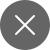# Understanding of Multi-Layer Perceptron(MLP)

3+

## Introduction

The core idea which gave inspiration to neural networks was the concept of biological neurons, the neurons present in the brain constitute the basic working of the neural network which are connected using dendrites. The individual neurons clubbed together gave rise to the idea of a multi-layered perceptron.

To understand a multilayer perceptron, one must have a clear understanding of neural networks and a perceptron. So it always raises the question of what is a neural network and what is a perceptron.

### Perceptron

Before understanding the concept of a multi-layer perceptron, we must first understand what a perceptron means. A perceptron is the most fundamental unit of a neural network, it takes in ‘n’ number of inputs from the various features known as “x” which are present in the input side, and with the help of the given weights ‘w’, we can use it to produce an output giving us the expected value. The output is then passed through an activation function, for example, a sigmoid function that is used to regularize the output value to be between 0 or 1 (the value if greater than 0 is 1 and if less than 0 is 0).

We then reiterate the entire process backward with the help of “back-propagation” which is used to rectify the errors of the training set outputs.The above figure represents the computational model of a single layer perceptron.

The input which is provided to the perceptron is represented with the help of an array which is x = [x1,x2,x3,…xn] which are the values representing the value of the pixels present in the image. As these are fed into the neuron, they are multiplied with the weights present in the layer which are the array consisting of the respective weights w = [w,w2,w3,…wn] which eventually develop the value of “z” also known as the “activation potential”. The extra term which we add here is known as the “bias”, the bias allows us to provide an extra degree of freedom to our model.

This value is then passed through an activation function σ as shown in the figure above. This activation function is used to limit the output value within a certain range and hereby generating the final output for the neuron. There are many activation layers, some of them include the sigmoid, tangent, hyperbolic, ReLU, and softmax.

### Multi-Layer Perceptron(MLP) in Machine Learning

Now that we have a firm understanding of the topic of a single layer perceptron, we now proceed to understand the concept of a multi-layered perceptron. The multi-layer perceptron is a type of network which is an accumulation of a group of neurons that are stacked together to form a layer and several of these layers are connected from a multi-layered perceptron.

In a multi-layered perceptron, the number of linear layers is more than one which is usually a combination of neurons as we discussed above.In simpler words, the MLP is nothing but a combination of a layer of perceptron interconnected together. The MLP uses the output which is provided by the previous layer or in this case, it would be the input layer and after passing the calculated values through the several layers (hidden layers) it reaches the output layer.

The main goal of MLP is to estimate some function f(). For example, the classifier which is mentioned above we see that it tries to use a mapping function(y = f(x)) to map the input x to the output y. The goal of this classifier becomes to learn this function and find out the best parameters required for the mapping function.

This process sums up the function for a single perceptron, but for a multi-layer perceptron, we know that these functions are chained together. So for a network with three layers, the mapping function would be f(x) = (f(3)f(2)(f(1)(x))). The layers which are present here perform a set of mathematical calculation and the end function would look like :

Y = f(W*x+ b) where “Y” is the output, “f” is the activation function, “W” is the set of weights and “x” is the input vector.

After this, we get an estimate of the output or the prediction which is used to define the loss function. The loss is determined by how far the predicted output is from the originally expected output.

### Feed Forward Network

A Feedforward network is one of the most commonly used and a typical example of the neural network. The target of a feed-forward network is to approximate the function f( ) which is used to calculate the output.

Feedforward network is a type of rebounding networks in which mostly there are two types of motions involved which are:

### The Forward Pass

The forward pass involves the input signal passing through the hidden layer, from the input layer and the output layer determines the measured value against the calculated value. The forward pass is essentially used to turn the input into the output.

### Back Propagation

The backpropagation step is an application of the chain rule of calculus and also involves finding the partial derivatives of the error functions which are calculated. The process of backpropagation mainly involves differentiation which helps us to derive a gradient that can be used to reduce the loss function. The state where the error is not present is known as convergence.3+

#### You may also like...